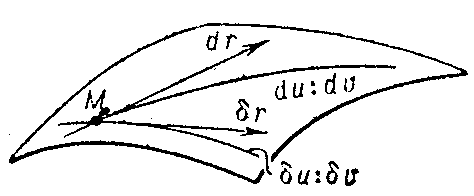# First fundamental form

metric form, of a surface

A quadratic form in the differentials of the coordinates on the surface that determines the intrinsic geometry of the surface in a neighbourhood of a given point.

Let the surface be defined by the equation

$$\mathbf r = \mathbf r ( u , v),$$

where $u$ and $v$ are coordinates on the surface, while

$$d \mathbf r = \mathbf r _ {u} du + \mathbf r _ {v} dv$$

is the differential of the position vector $\mathbf r$ along the chosen direction $du :dv$ of displacement from a point $M$ to an infinitesimally close point $M ^ \prime$( see Fig. a).Figure: f040450a

The square of the principal linear part of the increment of the length of the arc $MM ^ \prime$ can be expressed in terms of the square of the differential $d \mathbf r$:

$$\textrm{ I } = ds ^ {2} = d \mathbf r ^ {2} = \mathbf r _ {u} ^ {2} du ^ {2} + 2 \mathbf r _ {u} \mathbf r _ {v} du dv + \mathbf r _ {v} ^ {2} dv ^ {2} ,$$

and is called the first fundamental form of the surface. The coefficients in the first fundamental form are usually denoted by

$$E = \mathbf r _ {u} ^ {2} ,\ \ F = ( \mathbf r _ {u} , \mathbf r _ {v} ) ,\ \ G = \mathbf r _ {v} ^ {2} ,$$

or, in tensor symbols,

$$d \mathbf r ^ {2} = g _ {11} du ^ {2} + 2g _ {12} du dv + g _ {22} dv ^ {2} .$$

The tensor $g _ {ij}$ is called the first fundamental, or metric, tensor of the surface. The first fundamental form is a positive-definite form at regular points on the surface:

$$EG - F ^ { 2 } > 0.$$

The first fundamental form characterizes the metric properties of the surface: Knowledge of the first fundamental form enables one to calculate arc lengths on the surface:

$$s = \int\limits _ {t _ {0} } ^ { t } \sqrt {E \left ( \frac{du}{dt} \right ) ^ {2} + 2F \frac{du}{dt} \frac{dv}{dt} + G \left ( \frac{dv}{dt} \right ) ^ {2} } dt,$$

where $t$ is a parameter on the curve; angles between curves on the surface:

$$\cos ( \widehat{ {dr \delta r }} ) =$$

$$= \ \frac{E du \delta u + F( du \delta v+ dv \delta u)+ G dv \delta v }{\sqrt {E du ^ {2} + 2F du dv+ G dv ^ {2} } \sqrt {E \delta u ^ {2} + 2F \delta u \delta v+ G \delta v ^ {2} } } ,$$

where $du : dv$ and $\delta u : \delta v$ are the directions of the tangent vectors to the curves (see Fig. b); and areas of regions on the surface:

$$\sigma = \int\limits \int\limits \sqrt {EG - F ^ { 2 } } du dv .$$Figure: f040450b

The form of the coefficients of the first fundamental form substantially depends on the choice of the coordinates. The first fundamental form has so-called orthogonal form

$$E( u, v) du ^ {2} + G( u, v) dv ^ {2}$$

in orthogonal coordinates, canonical form

$$du ^ {2} + G ^ {2} dv ^ {2}$$

in semi-geodesic coordinates, and isothermal (isometric) form in isothermal coordinates:

$$ds ^ {2} = \lambda ^ {2} ( u, v)( du ^ {2} + dv ^ {2} ).$$

Sometimes a surface is characterized by special forms of the first fundamental form. For example, a Liouville surface is characterized by:

$$[ \phi ( u) + \psi ( v)]( du ^ {2} + dv ^ {2} ).$$

The first fundamental form is a bending invariant for the surface: The Gaussian curvature at a given point can be calculated from the coefficients of the first fundamental form and their derivatives only (Gauss' theorem).

See Fundamental forms of a surface for the relation between the first fundamental form and other quadratic forms, as well as for literature.

In more current terminology the first fundamental form of an imbedded surface $f: U \rightarrow \mathbf R ^ {3}$, $U \subset \mathbf R ^ {2}$, is defined as follows. The inclusion $( Tf ) _ {u} ( \mathbf R _ {u} ^ {2} ) \subset T _ {f ( u) } \mathbf R ^ {3}$ defines a quadratic form on the tangent space $( Tf ) _ {u} ( \mathbf R _ {u} ^ {2} )$ to the surface at $f ( u)$ by restricting the canonical scalar product on $T _ {f ( u) } \mathbf R ^ {3} \simeq \mathbf R ^ {3}$. Thus the first fundamental form of the surface $f ( U) \subset \mathbf R ^ {3}$ simply assigns to a pair of tangent vectors $X, Y$ to $f ( U)$ at $z \in f ( U)$ the inner product of $X$ and $Y$ in $\mathbf R ^ {3}$.